# 共享密钥加密与公开密钥加密

## 共享密钥加密

### 处理流程图解

• A使用密钥加密数据，并将秘文发送给B。
• B收到秘文后，使用相同的密钥对其进行解密。这样B就取得了原本的数据。此时的数据已经是加密好的了，就不需要担心第三者窃取数据了，因为它没有密钥解开此密文。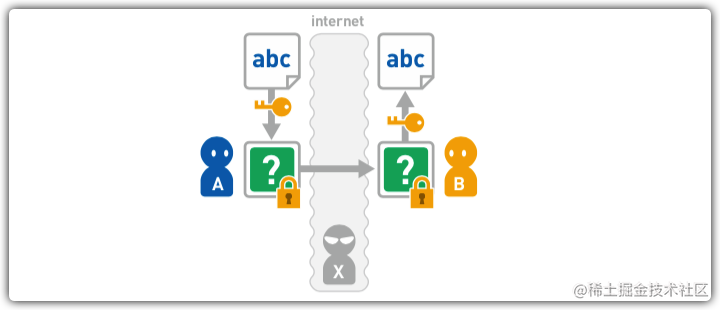### 可能产生的问题

• 此时，B不知道加密时使用的是什么密钥。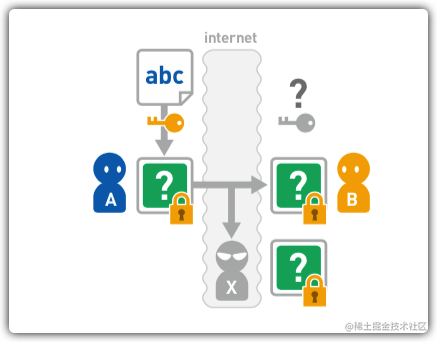• A需要通过某种手段将密钥交给B。和密文一样，A又在互联网上向B发送了密钥。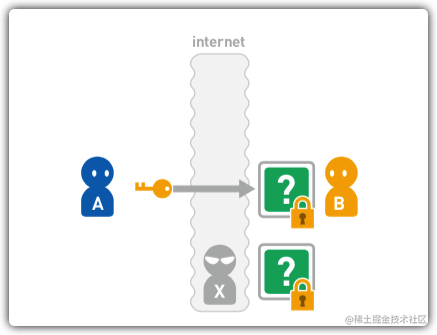• B使用收到的密钥对密文进行解密，但是该密钥也有可能会被X窃听，这样以来X也可以使用密钥对密文进行解密了。

### JAVA实现AES加密

• 创建AESUtils文件,编写AES加密工具类
``````package com.lk.util;

import javax.crypto.Cipher;
import javax.crypto.KeyGenerator;
import javax.crypto.SecretKey;
import java.io.Closeable;
import java.io.File;
import java.io.FileInputStream;
import java.io.FileOutputStream;
import java.io.IOException;
import java.io.InputStream;
import java.io.OutputStream;
import java.security.SecureRandom;

public class AESUtils {
/**
* 密钥长度: 128, 192 or 256
*/
private static final int KEY_SIZE = 128;

/**
* 加密/解密算法名称
*/
private static final String ALGORITHM = "AES";

/**
* 随机数生成器（RNG）算法名称
*/
private static final String RNG_ALGORITHM = "SHA1PRNG";

/**
* 生成密钥对象
* @param key byte[] 类型参数
* @return AES密钥对象
*/
private static SecretKey generateKey(byte[] key) throws Exception {
// 创建安全随机数生成器
SecureRandom random = SecureRandom.getInstance(RNG_ALGORITHM);
// 设置 密钥key的字节数组 作为安全随机数生成器的种子
random.setSeed(key);

// 创建 AES算法生成器
KeyGenerator gen = KeyGenerator.getInstance(ALGORITHM);
// 初始化算法生成器
gen.init(KEY_SIZE, random);

// 生成 AES密钥对象
return gen.generateKey();
}

/**
* 数据加密: 明文 -> 密文
*/
public static byte[] encrypt(byte[] plainBytes, byte[] key) throws Exception {
// 生成密钥对象
SecretKey secKey = generateKey(key);

// 获取 AES 密码器
Cipher cipher = Cipher.getInstance(ALGORITHM);
// 初始化密码器（加密模型）
cipher.init(Cipher.ENCRYPT_MODE, secKey);

// 加密数据, 返回密文

return cipher.doFinal(plainBytes);
}

/**
* 数据解密: 密文 -> 明文
*/
public static byte[] decrypt(byte[] cipherBytes, byte[] key) throws Exception {
// 生成密钥对象
SecretKey secKey = generateKey(key);

// 获取 AES 密码器
Cipher cipher = Cipher.getInstance(ALGORITHM);
// 初始化密码器（解密模型）
cipher.init(Cipher.DECRYPT_MODE, secKey);

// 解密数据, 返回明文
return cipher.doFinal(cipherBytes);
}

/**
* byte数组转16进制
*
* @param bytes byte数组
* @return 返回16进制字符串
*/
public static String bytesToHex(byte[] bytes) {
StringBuilder sb = new StringBuilder();
for (byte aByte : bytes) {
String hex = Integer.toHexString(aByte & 0xFF);
if (hex.length() < 2) {
sb.append(0);
}
sb.append(hex);
}
return sb.toString();
}

/**
* 16进制转byte
* @param inHex 16进制字符串
* @return byte
*/
public static byte hexToByte(String inHex) {
return (byte) Integer.parseInt(inHex, 16);
}

/**
* 16进制转byte数组
* @param inHex 16进制字符串
* @return byte数组
*/
public static byte[] hexToByteArray(String inHex) {
int hexlen = inHex.length();
byte[] result;
if (hexlen % 2 == 1) {
//奇数
hexlen++;
result = new byte[(hexlen / 2)];
inHex = "0" + inHex;
} else {
//偶数
result = new byte[(hexlen / 2)];
}
int j = 0;
for (int i = 0; i < hexlen; i += 2) {
result[j] = hexToByte(inHex.substring(i, i + 2));
j++;
}
return result;
}

/**
* 加密文件: 明文输入 -> 密文输出
*/
public static void encryptFile(File plainIn, File cipherOut, byte[] key) throws Exception {
aesFile(plainIn, cipherOut, key, true);
}

/**
* 解密文件: 密文输入 -> 明文输出
*/
public static void decryptFile(File cipherIn, File plainOut, byte[] key) throws Exception {
aesFile(plainOut, cipherIn, key, false);
}

/**
* AES 加密/解密文件
*/
private static void aesFile(File plainFile, File cipherFile, byte[] key, boolean isEncrypt) throws Exception {
// 获取 AES 密码器
Cipher cipher = Cipher.getInstance(ALGORITHM);
// 生成密钥对象
SecretKey secKey = generateKey(key);
// 初始化密码器
cipher.init(isEncrypt ? Cipher.ENCRYPT_MODE : Cipher.DECRYPT_MODE, secKey);

// 加密/解密数据
InputStream in = null;
OutputStream out = null;

try {
if (isEncrypt) {
// 加密: 明文文件为输入, 密文文件为输出
in = new FileInputStream(plainFile);
out = new FileOutputStream(cipherFile);
} else {
// 解密: 密文文件为输入, 明文文件为输出
in = new FileInputStream(cipherFile);
out = new FileOutputStream(plainFile);
}

byte[] buf = new byte;
int len = -1;

// 循环读取数据 加密/解密
while ((len = in.read(buf)) != -1) {
out.write(cipher.update(buf, 0, len));
}
out.write(cipher.doFinal());    // 最后需要收尾

out.flush();

} finally {
close(in);
close(out);
}
}

private static void close(Closeable c) {
if (c != null) {
try {
c.close();
} catch (IOException e) {
// nothing
}
}
}
}

• 在main函数中测试工具类
`````` public static void main(String[] args) throws Exception {
// 原文内容
String content = "你好，我是要发送的数据";

// AES加密/解密用的原始密码
String key = "MagicalProgrammer";

// 加密数据, 返回密文
byte[] cipherBytes = AESUtils.encrypt(content.getBytes(), key.getBytes());

// byte[]转16进制
String cipherString = AESUtils.bytesToHex(cipherBytes);

// 输出加密后的16进制密文
System.out.println("加密后的密文为: ");
System.out.println(cipherString);

// 解密数据, 返回明文
byte[] plainBytes = AESUtils.decrypt(AESUtils.hexToByteArray(cipherString), key.getBytes());

// 输出解密后的明文
System.out.println("解密后结果为: ");
System.out.println(new String(plainBytes));
}

## 公开密钥加密

### 处理流程图解

• 首先，由接收方B来生成公开密钥和私有密钥。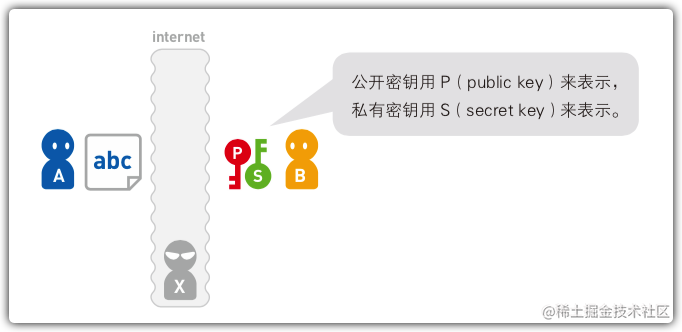• 然后，将公开密钥发送给B。• A使用B发来的公开密钥加密数据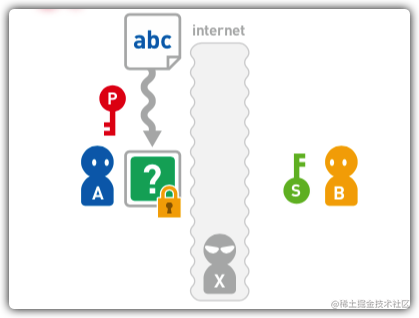• A将密文发送给B，B再使用私有密钥对密文进行解密。这样，B就得到了原本的数据。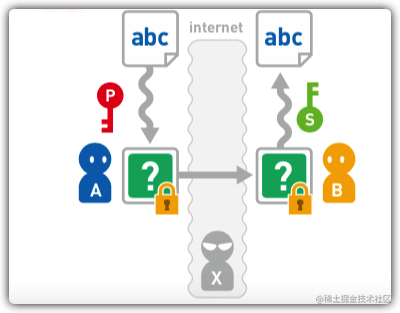• 公开密钥和密文都是通过互联网传输的，因此可能被X窃听。但是，使用公开密钥无法解密密文，因此X也无法得到原本的数据。

### 方便多人传输数据

• 首先，想发送数据的人需要从王山取得B发布的公开密钥。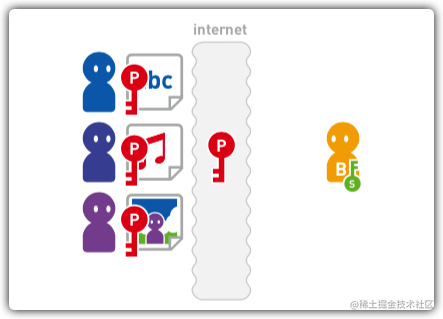• 然后，用获取到的公开密钥加密要发送的数据。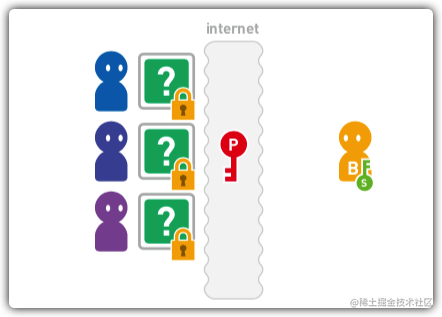• 最后，把密文发送给B• B用私有密钥对收到的密文进行解密，取得原本的数据。这种情况就不需要为每个发送对象都准备对应的密钥了。需要保密的私有密钥由接收方保管，所以安全性也更高。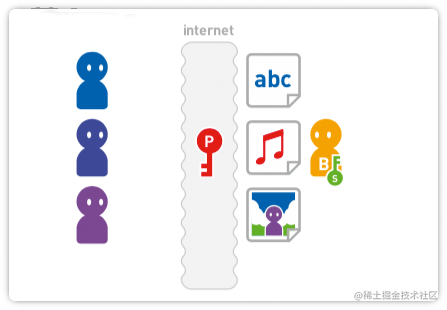### 中间人攻击

X想要窃听A发送给B的数据，于是他准备了公开密钥PX和私有密钥SX。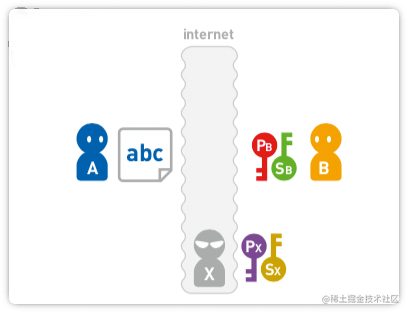• 在B把公开密钥PB发送给A的时候• X把公开密钥PB替换成自己的PX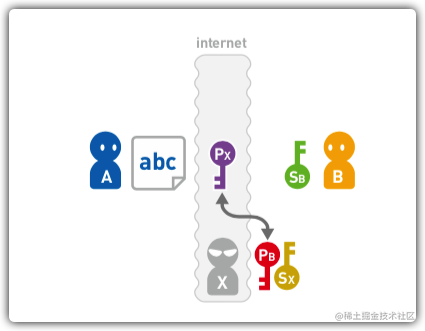• 于是公开密钥Px传到了A那里，由于公开密钥无法显示自己是由谁生成的，所以A不会发现自己收到的公开密钥已经被人替换。• A使用公开密钥PX对数据加密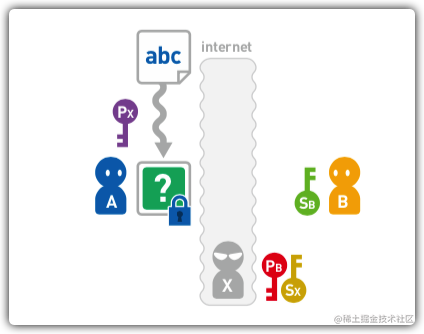• 当A把想要给B的密文发送出去后，X接收了这个密文。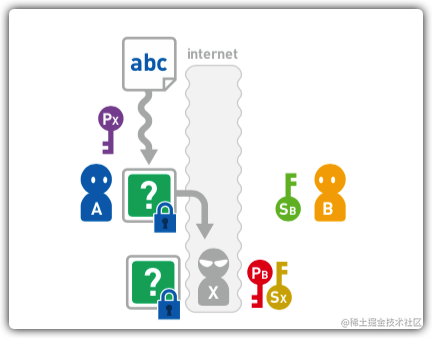• 这个密文由X生成的公开密钥PX加密而成，所以X可以用自己的私有密钥SX对密文进行解密。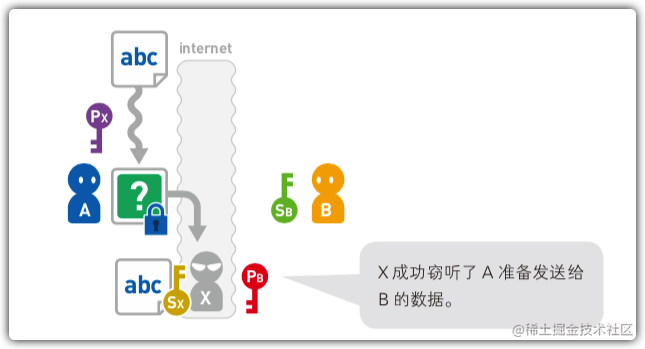• X用B生成的公开密钥PB加密数据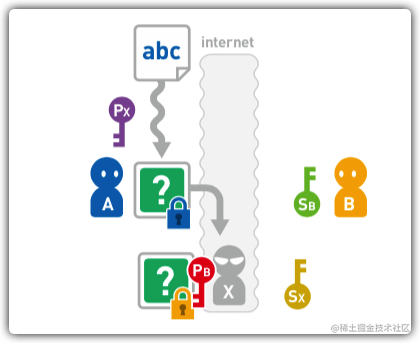• X把密文发送给B，这个密文由B发出的公开密钥PB加密而成，所以B可以用自己的私有密钥SB来解密，从收到密文到解密密文都没发生任何问题，因此B也不可能意识到自己已经被窃听。### 实现难点

• 可以使用某个数值对数据进行加密
• 使用另一个数值对加密数据进行计算就可以让数据恢复原样。
• 无法从一种密钥推算出另一种密钥。

### JAVA实现RSA加密

• 创建RSAUtils文件，编写RSA加密工具类
``````package com.lk.util;

import java.util.Base64;
import javax.crypto.Cipher;
import java.security.KeyFactory;
import java.security.KeyPair;
import java.security.KeyPairGenerator;
import java.security.NoSuchAlgorithmException;
import java.security.SecureRandom;
import java.security.interfaces.RSAPrivateKey;
import java.security.interfaces.RSAPublicKey;
import java.security.spec.PKCS8EncodedKeySpec;
import java.security.spec.X509EncodedKeySpec;
import java.util.HashMap;
import java.util.Map;

/**
* RSA加密工具类
*/
public class RSAUtils {
/**
* 密钥长度 于原文长度对应 以及越长速度越慢
*/
private final static int KEY_SIZE = 1024;
/**
* 用于封装随机产生的公钥与私钥
*/
private static Map<Integer, String> keyMap = new HashMap<Integer, String>();

/**
* 随机生成密钥对
*/
public static Map genKeyPair() throws NoSuchAlgorithmException {
// KeyPairGenerator类用于生成公钥和私钥对，基于RSA算法生成对象
KeyPairGenerator keyPairGen = KeyPairGenerator.getInstance("RSA");
// 初始化密钥对生成器
keyPairGen.initialize(KEY_SIZE, new SecureRandom());
// 生成一个密钥对，保存在keyPair中
KeyPair keyPair = keyPairGen.generateKeyPair();
// 得到私钥
RSAPrivateKey privateKey = (RSAPrivateKey) keyPair.getPrivate();
// 得到公钥
RSAPublicKey publicKey = (RSAPublicKey) keyPair.getPublic();
String publicKeyString = Base64.getEncoder().encodeToString(publicKey.getEncoded());
// 得到私钥字符串
String privateKeyString = Base64.getEncoder().encodeToString(privateKey.getEncoded());
// 将公钥和私钥保存到Map
//0表示公钥
keyMap.put(0, publicKeyString);
//1表示私钥
keyMap.put(1, privateKeyString);
return keyMap;
}

/**
* RSA公钥加密
*
* @param str       加密字符串
* @param publicKey 公钥
* @return 密文
* @throws Exception 加密过程中的异常信息
*/
public static String encrypt(String str, String publicKey) throws Exception {
//base64编码的公钥
byte[] decoded = Base64.getDecoder().decode(publicKey);
RSAPublicKey pubKey = (RSAPublicKey) KeyFactory.getInstance("RSA").generatePublic(new X509EncodedKeySpec(decoded));
//RSA加密
Cipher cipher = Cipher.getInstance("RSA");
cipher.init(Cipher.ENCRYPT_MODE, pubKey);
String outStr = Base64.getEncoder().encodeToString(cipher.doFinal(str.getBytes("UTF-8")));
return outStr;
}

/**
* RSA私钥解密
*
* @param str        加密字符串
* @param privateKey 私钥
* @return 明文
* @throws Exception 解密过程中的异常信息
*/
public static String decrypt(String str, String privateKey) throws Exception {
//64位解码加密后的字符串
byte[] inputByte = Base64.getDecoder().decode(str);
//base64编码的私钥
byte[] decoded = Base64.getDecoder().decode(privateKey);
RSAPrivateKey priKey = (RSAPrivateKey) KeyFactory.getInstance("RSA").generatePrivate(new PKCS8EncodedKeySpec(decoded));
//RSA解密
Cipher cipher = Cipher.getInstance("RSA");
cipher.init(Cipher.DECRYPT_MODE, priKey);
String outStr = new String(cipher.doFinal(inputByte));
return outStr;
}
}

• 在main函数中测试工具类
``````public static void main(String[] args) throws Exception {
// 随机生成的密钥和公钥
Map<Integer, String> keyMap = new HashMap<Integer, String>();
//生成公钥和私钥
keyMap = RSAUtils.genKeyPair();
System.out.println("公钥:" + keyMap.get(0));
System.out.println("私钥:" + keyMap.get(1));

// 要加密的数据
String message = "你好，我是通过RSA加密的数据";

// 使用公钥加密
String messageEn = RSAUtils.encrypt(message, keyMap.get(0));
System.out.println("密文:" + messageEn);

// 使用私钥解密
String messageDe = RSAUtils.decrypt(messageEn, keyMap.get(1));
System.out.println("解密:" + messageDe);
}

## 写在最后

• 文中使用的图片源自《我的第一本算法书》，如若侵权，请评论区留言，作者立即删除相关图片。
• 文中如有错误，欢迎在评论区指正，如果这篇文章帮到了你，欢迎点赞和关注😊
• 本文首发于掘金，未经许可禁止转载💌